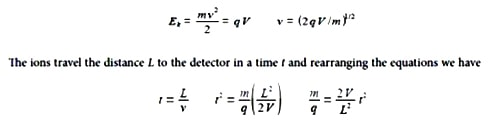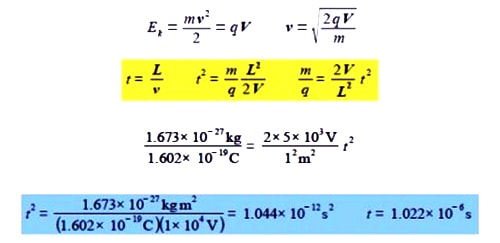Chemistry

# Time of Flight Mass Spectrometer

A time of flight (TOF) mass spectrometer is sketched below-The TOF MS is all under a vacuum. A pulsed laser fires a pulse of light onto a sample plate causing a pulse of ions of mass m and charges q to be ejected from the sample (q = ze the number of charges z and e the elementary charge). The ions are accelerated by a voltage V applied between the sample plate and the flight tube. The flight tube is of length L (typically 1 meter) with no electric or magnetic fields in it. The pulse of ions leave the flight tube and strikes the detector. The electric field V gives the ions a kinetic energy Ek, with a velocity v of the ions in the flight tube.As the voltage V and the flight tube length L are fixed, the value of m/q is directly related to the square of the time of flight. Firstly, rewrite these equations in vector notation. Secondly, if L = 1 m, and V = 5 kV, find the time of flight of a H+ ion of mass 1.673×10-27 kg and charge 1.602×10-19 C down the flight tube (be careful with the units). The flight time in the short acceleration region may be safely ignored.

SolutionWork = force x distance and so V C = J = N m but also force = massxacceleration = kg m s-2 and so V C = J = kg m2 s-2. The proton has a flight time of about a microsecond.